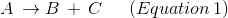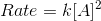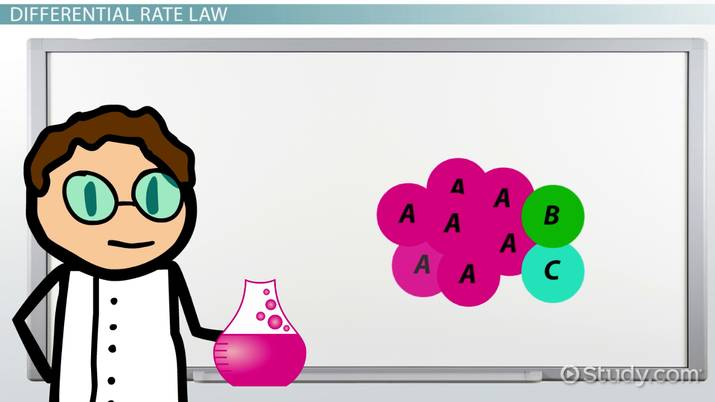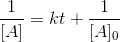# Second Order Integrated Rate Law

Lesson Transcript
Instructor: LaRita Williams

LaRita holds a master's degree and is currently an adjunct professor of Chemistry.

In this lesson, we will give the equation for the second order integrated rate law. We'll discuss its graph and then set up and solve a simple example problem.

## Differential Rate Law

Today's technology makes it possible for almost anything to happen fast. Breaking news can be at your fingertips with the click of a button. Full meals can be microwaved in a just a couple of minutes. Cross-country travel might only take a few short hours. In a society obsessed with getting things done quickly, why not also be concerned with the speed of your chemical reactions? The investigation of kinetics allows us to do just that. Kinetics is the study of the rate at which chemical reactions occur.

In kinetics, reactions are classified according to their order. The order of a reaction is the experimentally determined exponent to which each reactant concentration must be raised in the differential rate law equation. If a reaction is second order with respect to a certain reactant, then that reactant is being raised to a power of 2 in its differential rate law equation.

For the generic reaction you can see below (Equation 1), where reactant A goes to products B and C, the differential rate law is given. Note, that in the differential rate law equation, the concentration of reactant A is raised to a power 2, indicating that this reaction is second order with respect to A.The differential rate law models how the rate of a reaction depends on the concentrations of species in that reaction. On the other hand, the integrated rate law shows how the concentrations of species in a reaction depend on time.An error occurred trying to load this video.

Try refreshing the page, or contact customer support.

Coming up next: State Functions in Thermochemistry

### You're on a roll. Keep up the good work!

Replay
Your next lesson will play in 10 seconds
• 0:00 Differential Rate Law
• 1:33 Second Order…
• 2:10 How to Graph the Equation
• 2:46 An Example Calculation
• 3:56 Lesson Summary
Save Save

Want to watch this again later?

Timeline
Autoplay
Autoplay
Speed Speed

## Second Order Integrated Rate Law Equation

An integration of the second order differential rate law that we looked at a moment ago gives us the second order integrated rate law equation, shown on your screen below:In this equation, Asub0 represents the initial concentration of reactant A; t is the variable for time; k is the rate constant of the reaction, and A represents the concentration of reactant A at time, t. If the values of k and Asub0 are known, we can calculate the concentration of A at any given time, t, thus modeling how the concentration of that reactant is affected by time.

## How to Graph the Equation

Look carefully, and you'll notice that the equation for the second order integrated rate law is in the form y = mx +b, where y = 1/A; m = k; x = t; and b = 1/Asub0. We learned in other lessons that y = mx +b is the equation for a line. So, if we plot our x and y coordinates from the equation (t and 1/A), we will produce the graph of a straight line. Moreover, the slope of that line (m) will be equal to the rate constant of our reaction (k).

To unlock this lesson you must be a Study.com Member.

### Register to view this lesson

Are you a student or a teacher?